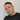# Calculating cumulative contribution in Excel

June 15, 2019

In this example again we’ll look at some order data:

Here we see a table of orders, grand total for each order and two as of yet empty colums that will hold the cumulative total contribution of each order as well as the cumulative percentage each order adds to the total.
To calculate the cumulative total we are going to use a combination of the SUM function and ranges, so in cell C2, type (and hit enter):

``=SUM(\$B\$2:B2)``

Notice the first cell reference was made static by hitting F4. Hitting enter on the formula fills the whole column and notice that the second cell reference is dynamic, which leads to range increasing incrementally for each row.

Now let’s look at the cumulative percentage of the total. In cell D2, type the following (and hit enter):

``=C2/(SUM([GRAND TOTAL (€)]))  ``

Where the ‘GRAND TOTAL (€)’ is a reference to the column that holds the order totals (you could just as well use the cell ranges here). Since we are basing this calculation based off the values achieved in the previous calculation we can take advantage of the incremental approach in that case and use dynamic cell references here.

To get the values formated as percent in the last column, just select it and hit the percentage button in upper right corner.Written by Johan Osterberg who lives and works in Gothenburg, Sweden as a developer specialized in e-commerce. Connect with me on Linkedin